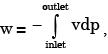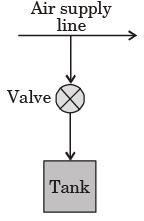Courses

# Past Year Questions: First Law Of Thermodynamics, Heat, Work And Energy Interactions Notes | EduRev

## Mechanical Engineering : Past Year Questions: First Law Of Thermodynamics, Heat, Work And Energy Interactions Notes | EduRev

The document Past Year Questions: First Law Of Thermodynamics, Heat, Work And Energy Interactions Notes | EduRev is a part of the Mechanical Engineering Course Thermodynamics.
All you need of Mechanical Engineering at this link: Mechanical Engineering

Question 1:A gas is heated in a duct as it flows over a resistance heater. Consider a 101 kW electric heating system. The gas enters the heating section of the duct at 100 kPa and 27°C with a volume flow rate of 15 m3/s. If heat is lost from the gas in the duct to the surroundings at a rate of 51 kW, the exit temperature of the gas is (Assume constant pressure, ideal gas, negligible change in kinetic and potential energies and constant specific heat : Cp = 1 kJ/kg. K; R = 0.5 kJ/kg K).

[2019 , Set 1]

Question 2:A calorically perfect gas (specific heat at constant pressure 1000 J/kgK) enters and leaves a gas turbine with the same velocity.The emperature of the gas at turbine entry and exit are 1100 K and 400 K, respectively. The power produced is 4.6 MW and heat escapes at the rate of 300 kJ/s through the turbine casing.The mass flow rate of the gas (in kg/s) through the turbine is

[2017, Set-2]

Question 3:A compressor undergoes a reversible, steady flow process. The gas at inlet and outlet of the compressor is designated as state 1 and state 2 respectively. Potential and kinetic energy changes are to be ignored. The following notations are used: v = specific volume and P = pressure of the gas. The specific work required to be supplied to the compressor for this gas compression process is



Question 4:In a steady state steady flow process taking place in a device with a single inlet and a single outlet, the work done per unit mass flow rate is given bywhere v is the specific volume and p is the pressure, The expression for w given above



Question 5:A rigid, insulated tank is initially evacuated.
The tank is connected with a supply line through which air (assumed to be ideal gas with constant specific heats) passes at 1 MPa, 350°C.A valve connected with the supply line is opened and the tank is charged with air until the final pressure inside the tank reaches 1 MPa. The final temperature inside the tank



Question 6:A small steam whistle (perfectly insulated and doing no shaft work) causes a drop of 0.8 kJ/kg in enthalpy of steam from entry to exit. If the kinetic energy of the steam at entry is negligible, the velocity of the steam at exit is



Question 7:When an ideal gas with constant specific heats is throttled adiabatically, with negligible changes in kinetic and potential energies.



Question 8:A steam turbine receives steam steadily at 10 bar with a enthalpy of 3000 kJ/kg and discharges at 1 bar with an enthalpy of 2700 kJ/kg. The work output is 250 kJ/kg. The changes in kinetic and potential energies are negligible. The heat transfer from the turbine casing to the surroundings is equal to



Question 9:For reversible a diabatic compression in a steady flow process, the work transfer per unit mass is



Question 10:The contents of a well-insulated tank are heated by a resistor of 23 W in which 10 A current is flowing. Consider the tank along with its contents as a thermodynamic system. The work done by the system and the heat transfer to the system are positive. The rates of heat (Q), work (W) and change in internal energy (ΔU) during the process (kW) are



Question 11:A gas expands in a frictionless piston-cylinder arrangement. The expansion process is very slow, and is resisted by an ambient pressure of 100 kPa. During the expansion process, the pressure of the system (gas) remains constant at 300 kPa. The change in volume of the gas is 0.01 m3. The maximum amount of work that could be utilized from the above process is.



Question 12:Which of the following relationships is valid only for reversible processes undergone by a closed system of simple compressible substance (neglect changes in kinetic and potential energy)?



Question 13:Gauge pressure of air to which the ball must have been originally inflated so that it would be equal 1 bar gauge at the stadium is



Question 14:The amount of heat lost by the air in the football and the gauge pressure of air in the football at the stadium respectively equal



Question 15:A 2 kW, 40 litres water heater is switched on for 20 minutes. The heat capacity cp for water is 4.2 kJ/kgK. Assuming all the electrical energy has gone into heating the water, increase of the water temperature in degree centigrade is



Question 16:The first law of thermodynamics takes the form W = –ΔH when applied to



Question 17:The internal energy of an ideal gas is a function of

[2016 , Set-2]

Question 18:For an ideal gas with constant values of specific heats, for calculation of the specific enthalpy,

[2015, Set-1]

Question 19:A frictionless piston-cylinder device contains a gas initially at 0.8 MPa and 0.015 m3. It expands quasistatically at constant temperature to a final volume of 0.030 m3. The work output (in kJ) during this process will be



Question 20:A balloon containing an ideal gas is initially kept in an evacuated and insulated room. The balloon ruptures and the gas fills up the entire room. Which one of the following statements is TRUE at the end of above process?



Offer running on EduRev: Apply code STAYHOME200 to get INR 200 off on our premium plan EduRev Infinity!

## Thermodynamics

56 videos|92 docs|33 tests

,

,

,

,

,

,

,

,

,

,

,

,

,

,

,

,

,

,

,

,

,

,

,

,

,

,

,

;